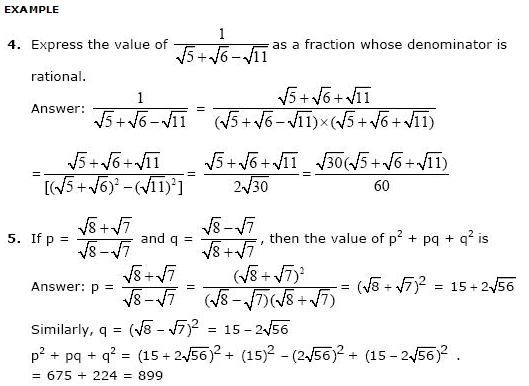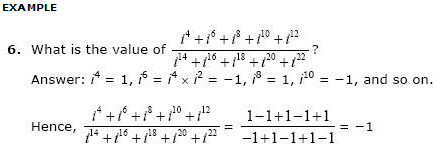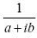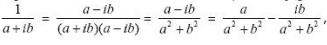# Types of Numbers, Number Theory, Quantitative Aptitude Notes | Study Quantitative Reasoning for GMAT - GMAT

## GMAT: Types of Numbers, Number Theory, Quantitative Aptitude Notes | Study Quantitative Reasoning for GMAT - GMAT

The document Types of Numbers, Number Theory, Quantitative Aptitude Notes | Study Quantitative Reasoning for GMAT - GMAT is a part of the GMAT Course Quantitative Reasoning for GMAT.
All you need of GMAT at this link: GMAT

Natural Numbers:
The group of numbers starting from 1 and including 1, 2, 3, 4, 5, and so on. Zero, negative numbers, and decimals are not included this group.

Whole Numbers:

All Natural Numbers plus the number 0 are called as Whole Numbers.

Integers:
All Whole Numbers and their negatives are included in this group.

Rational Numbers:
Any number that can be expressed as a ratio of two integers is called a rational number.

This group contains decimal that either do not exist (as in 6 which is 6/1), or terminate (as in 3.4 which is 34/10), or repeat with a pattern (as in 2.333... which is 7/3).

Irrational Numbers:

Any number that can not be expressed as the ratio of two integers is called an irrational number (imaginary or complex numbers are not included in irrational numbers).

These numbers have decimals that never terminate and never repeat with a pattern.Real Numbers:
This group is made up of all the Rational and Irrational Numbers. The ordinary number line encountered when studying algebra holds real numbers.

Imaginary Numbers:
An imaginary number is one of the form bi where b is a real number and i is the imaginary element derived from i2 = (-1). Imaginary numbers were defined to enable the solutions of equations requiring the square roots of negative numbers.Complex Numbers:
A Complex Numbers is a combination of a real number and an imaginary number in the form a + bi. a is called the real part and b is called the imaginary part.
Examples include 3 + 6i

Note:
A number in the formis written in the form of a complex number by a+lb multiplying both numerator and denominator by the conjugate of a + ib, i.e. a - ib. Hence,which is in the form p + iq

Prime Numbers:
All the numbers that have only two divisors, 1 and the number itself, are called prime numbers. Hence, a prime number can only be written as the product of 1 and itself. The numbers 2, 3, 5, 7, 11, 37, etc. are prime numbers.

Note:

1 is not a prime number.

EXAMPLE
Ques 1: If x2 - y2 = 101, find the value of x2 + y2, given that x and y are natural numbers.
Ans: x2 - y2 = (x + y)(x - y) = 101. But 101 is a prime number and cannot be written as product of two numbers unless one of the numbers is 1 and the other is 101 itself.

Hence, x + y = 101 and x - y = 1. -> x = 51, y = 50.

-> x2 + y2 = 512 + 502 = 5101.

Ques 2: What numbers have exactly three divisors?
Ans: The squares of prime numbers have exactly three divisors, i.e. 1, the prime number, and the square itself.
To find whether a number N is prime or not
Find the root R (approximate) of the number N, i.e. R = √N. Divide N by every prime number less than or equal to R. If N is divisible by at least one of those prime numbers it is not a prime number. If N is not divisible by any of those prime numbers, it is a prime number.

Odd and Even Numbers:
All the numbers divisible by 2 are called even numbers whereas all the numbers not divisible by 2 are called odd numbers. 2, 4, 6, 8 etc. are even numbers and 1, 3, 5, 7.. etc. are odd numbers.

Remember!
• Odd + Odd = Even
• Even + Even = Even
• Odd + Even = Odd
• (Odd)Even = Odd
• (Even)Odd = Even
• Even x Odd = Even
• Even x Even = Even
• Odd x Odd = Odd
• (Odd)Even x (Even)Odd = Even
• (Odd)Even + (Even)Odd = Odd

The document Types of Numbers, Number Theory, Quantitative Aptitude Notes | Study Quantitative Reasoning for GMAT - GMAT is a part of the GMAT Course Quantitative Reasoning for GMAT.
All you need of GMAT at this link: GMATUse Code STAYHOME200 and get INR 200 additional OFF

## Quantitative Reasoning for GMAT

75 videos|87 docs|156 tests

Track your progress, build streaks, highlight & save important lessons and more!

,

,

,

,

,

,

,

,

,

,

,

,

,

,

,

,

,

,

,

,

,

,

,

,

,

,

,

;